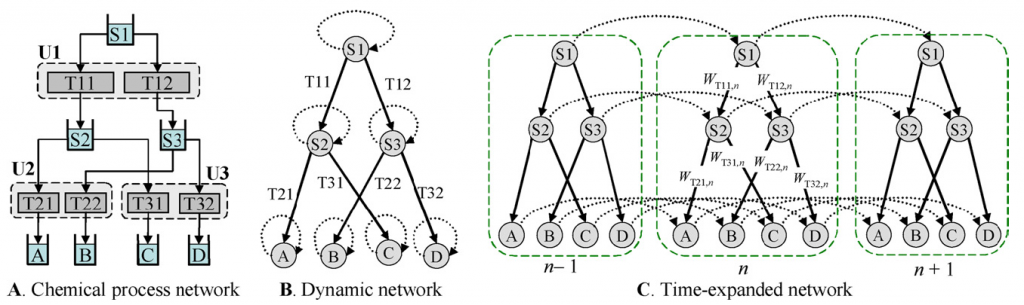# Theory

Since solving MIP scheduling models can be computationally expensive, we study the structure of MIP models to derive combinatorial insights for these formulation that would allow us to develop better solution methods. We investigate how chemical manufacturing facilities can be represented as dynamic networks and then converted into time-expanded networks with side constraints (Maravelias, 2012) (see Figure 1).Figure 1. Representation of chemical facility as a dynamic network and then time-expanded network. The assignment constraints are written as the side constraints in the time-expanded network.

One of the common characteristics in chemical processes is the presence of sequence-dependent changeover times. Unfortunately, accounting for changeovers leads to the MIP models that are computationally expensive. To address this issue, we propose five new formulations for sequence-dependent changeovers. In terms of theory, we first prove nine propositions regarding the relative tightness of different formulations (see Figure 2). Second, we prove that one of the formulations, (SIIT), is facet-defining for the problem that is subject to changeover and unit availability constraints. In terms of computation, some of the new formulations outperform the formulations in literature. Interestingly, we find that tighter formulations do not always lead to faster solution times (Velez et al., 2017).Figure 2. The relative tightness of sequence-dependent changeover formulations. White indicates that the formulation on the left is tighter than the formulation on the top; gray indicates the opposite. A dotted block indicates that neither formulation is tighter. Each letter(s) represent different changeover formulations: (K)/(KB) – Kondili et al. (1993), (SH) – Shah et al. (1993), (W) – Wolsey (1997), (SI)/(SII)/(SIII)/(SIIT)/(P) – Proposed formulation).

Reference:

Maravelias, C. T. (2012) On the combinatorial structure of discrete-time MIP formulations for chemical production scheduling. Computers & Chemical Engineering. 38, 204-212.

Velez, S., Dong, Y. and Maravelias, C. T. (2017) Changeover Formulations for Discrete-Time Mixed-integer Programming Scheduling Models.